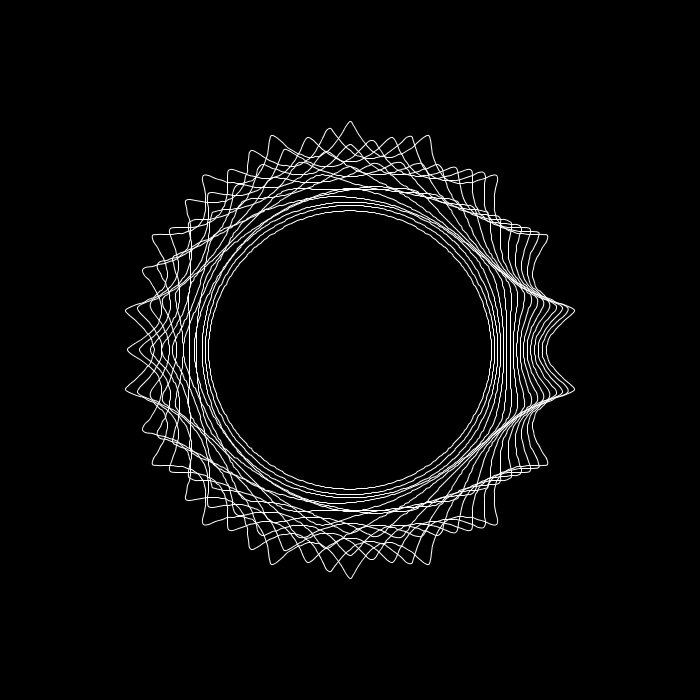A processing sketch can be simplified in JRubyArt

``````# Visualize: Superformula
# from Form+Code in Design, Art, and Architecture
# by Casey Reas, Chandler McWilliams, and LUST
# Princeton Architectural Press, 2010
# ISBN 9781568989372
#

attr_reader :scaler, :m, :n1, :n2, :n3

def settings
size(700, 700)
smooth(4)
end

def setup
sketch_title('Superformula')
no_fill
stroke(255)
@scaler = 200.0
@m = 2
@n1 = 18.0
@n2 = 1.0
@n3 = 1.0
end

def draw
background(0)
push_matrix
translate(width / 2, height / 2)
newscaler = scaler
16.downto(0) do |s|
begin_shape
mm = m + s
nn1 = n1 + s
nn2 = n2 + s
nn3 = n3 + s
newscaler *= 0.98
sscaler = newscaler
points = superformula(mm, nn1, nn2, nn3)
curve_vertex(
points[points.length - 1].x * sscaler,
points[points.length - 1].y * sscaler
)
(0...points.length).each do |i|
curve_vertex(points[i].x * sscaler, points[i].y * sscaler)
end
curve_vertex(points.x * sscaler, points.y * sscaler)
end_shape
end
pop_matrix
end

def superformula(m, n1, n2, n3)
num_points = 360
phi = TWO_PI / num_points
(0..num_points).map { |i| superformula_point(m, n1, n2, n3, phi * i) }
end

def superformula_point(m, n1, n2, n3, phi)
t1 = cos(m * phi / 4)
t1 = t1.abs
t1 **= n2
t2 = sin(m * phi / 4)
t2 = t2.abs
t2 **= n3
r = (t1 + t2)**(1 / n1)
return Vec2D.new if r.abs.zero?
x = cos(phi) / r
y = sin(phi) / r
Vec2D.new(x, y)
end
``````

### What’s it look like